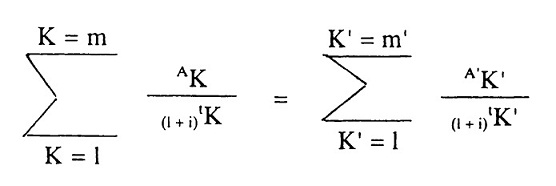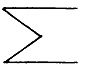# Consumer Credit Act, 1995 Permanent Page URL

FOURTH SCHEDULE

APR—Method of Calculation

THE BASIC EQUATION EXPRESSING THE EQUIVALENCE OF LOANS ON THE ONE HAND, AND REPAYMENTS AND CHARGES ON THE OTHER:Meaning of letters and symbols: K is the number of a loan K′ is the number of a repayment or a payment of charges AK is the amount of loan number K A′K′ is the amount of repayment number K′represents a sum m is the number of the last loan m′ is the number of the last repayment or payment of charges tK is the interval, expressed in years and fractions of a year, between the date of loan No. 1 and those of subsequent loans Nos. 2 to m′ tK′ is the interval expressed in years and fractions of a year between the date of loan No. 1 and those of repayments or payments of charges Nos. 1 to m1 i is the percentage rate that can be calculated (either by algebra, by successive approximations, or by a computer programme) where the other terms in the equation are known from the contract or otherwise.

Remarks

(a) The amounts paid by both parties at different times shall not necessarily be equal and shall not necessarily be paid at equal intervals.

(b) The starting date shall be that of the first loan.

(c) Intervals between dates used in the calculations shall be expressed in years or in fractions of a year.